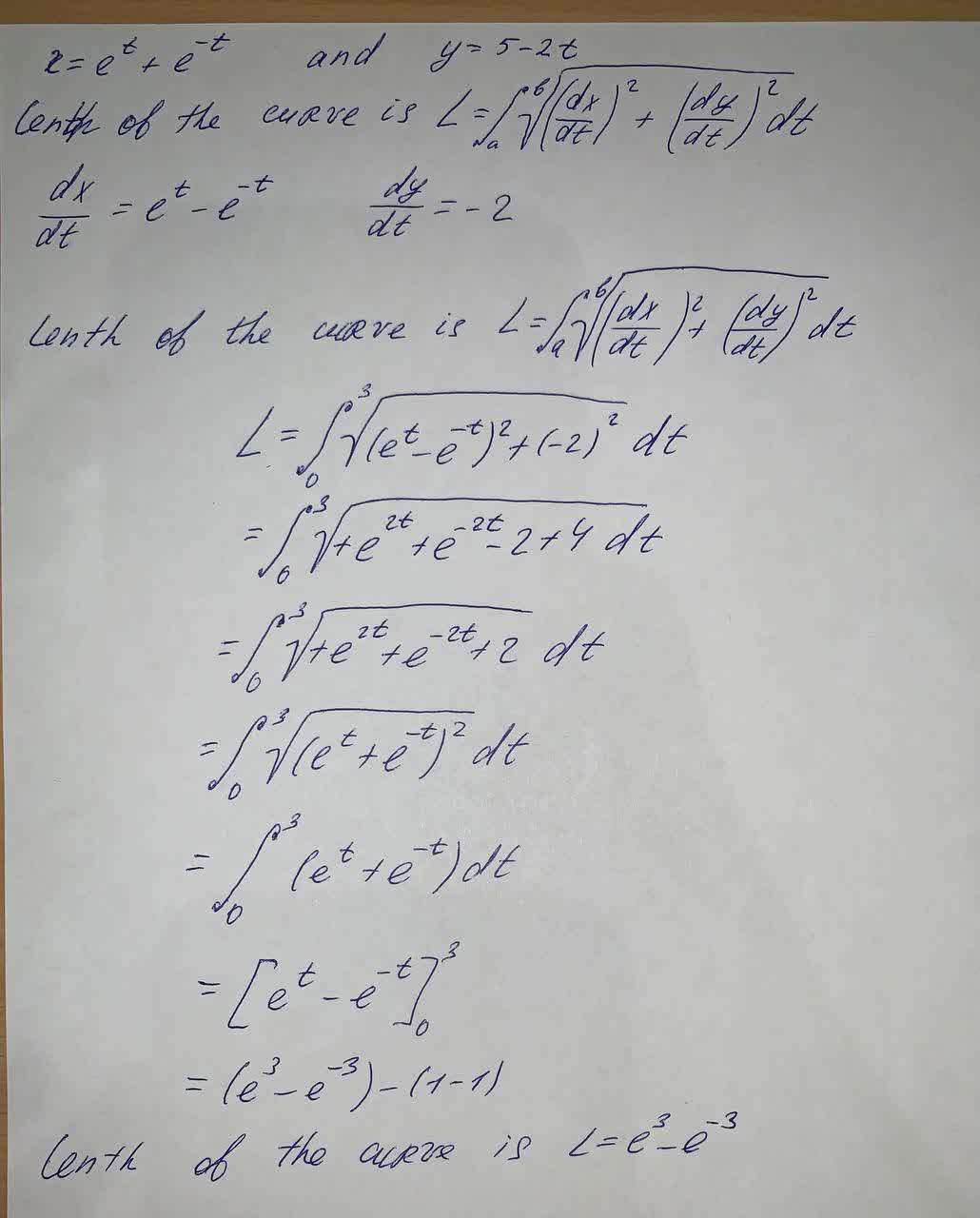# Find the Exact length of the curve. x=e^t+e^{-t} , y=5-2t betweenCarol Gates 2021-09-11 Answered
Find the Exact length of the curve.
$x={e}^{t}+{e}^{-t},y=5-2t$ between $0\le t\le 3$
You can still ask an expert for help

## Want to know more about Laplace transform?

• Questions are typically answered in as fast as 30 minutes

Solve your problem for the price of one coffee

• Math expert for every subject
• Pay only if we can solve itFaiza Fuller
To find: